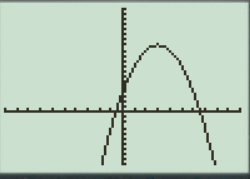# To graph : The quadratic equation y = 4 + 6 x − x 2 in a graphing window.### Precalculus: Mathematics for Calcu...

6th Edition
Stewart + 5 others
Publisher: Cengage Learning
ISBN: 9780840068071### Precalculus: Mathematics for Calcu...

6th Edition
Stewart + 5 others
Publisher: Cengage Learning
ISBN: 9780840068071

#### Solutions

Chapter 1.9, Problem 13E
To determine

## To graph: The quadratic equation y=4+6x−x2 in a graphing window.

Expert Solution

### Explanation of Solution

Given information:

Graph:

The graph of the quadratic equation y=4+6xx2 can be sketched in the cartesian plane,

Consider the quadratic equation, y=4+6xx2 .

Rewrite the equation:

y=4+6xx2y=x2+6x+4

Solve the equation y=x2+6x+4 with the quadratic formula:

b±b24ac2a

Now solve the equation y=x2+6x+4 by quadratic formula:

Find the value of a,b,c :

The value of a,b,c are , a is the coefficient of x2 , b is the coefficient of x , c is constant.

Thus, the value of:

a=1b=6c=4

Substitute the values of a,b,c in quadratic formula:

b±b24ac2a

b±b24ac2a=6±62×1×42×1=±36+162=±6+42=±102

Either the value ±102 is positive or negative:

When the value ±102 is positive, then the real root is 5 ,

When the value ±102 is negative, then the real root is 5 ,

So x=5 or

x=5 ,

Here observed the equation of parabola, the coefficient of x2 is negative, so the graph is opened downward.

The roots and the shape of parabola is cleared.

The graph of the equation y=4+6xx2 .

Substitute x=0 , in the equation y=x2+6x+4 ,

y=4+6xx2y=x2+6x+4y=02+60+4y=4

Substitute x=1 , in the equation y=x2+6x+4 ,

y=x2+6x+4y=(1)2+61+4y=1+6+4y=9

Substitute x=2 , in the equation y=x2+6x+4 ,

y=x2+6x+4y=(2)2+62+4y=4+12+4y=12

Observe that as the value of x increases there is the decrement in value of y .

Steps to plot the graph of the equation y=x2+6x+4 with the help of graphing utility are as follows:

Step 1: Press MODE key.

Step 2: Use the down arrow key to reach FUNC option.

Step 3: Press ENTER key.

Step 4: Press Y= key.

Step 5: Enter the function y=x2+6x+4 .

Step 6: Press WINDOW key. Change the settings to

Xmin=10Xmax=10Ymin=10Ymax=20

For better view of graph.

Step 8: Press GRAPH key.

The result obtained on the screen is provided below,Interpretation:

The equation of the function y=x2+6x+4 represents a parabola.

The parabola opens downward.

The x intercepts are the points on xaxis where the graph of the equation touches xaxis .

The equation has two real roots.

The coefficients of x2 is negative so the graph of the equation open downward.

Therefore value of x increases then a slightly increment comes in the value of y .

Therefore, the equation y=x2+6x+4 is symmetric about the y axis Initially the graph of the equation y=x2+6x+4 decreases when increase the value of x ,the graph is a parabola shaped graph.

### Have a homework question?

Subscribe to bartleby learn! Ask subject matter experts 30 homework questions each month. Plus, you’ll have access to millions of step-by-step textbook answers!